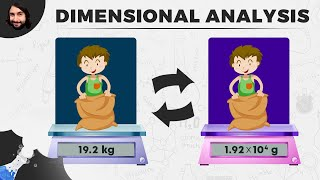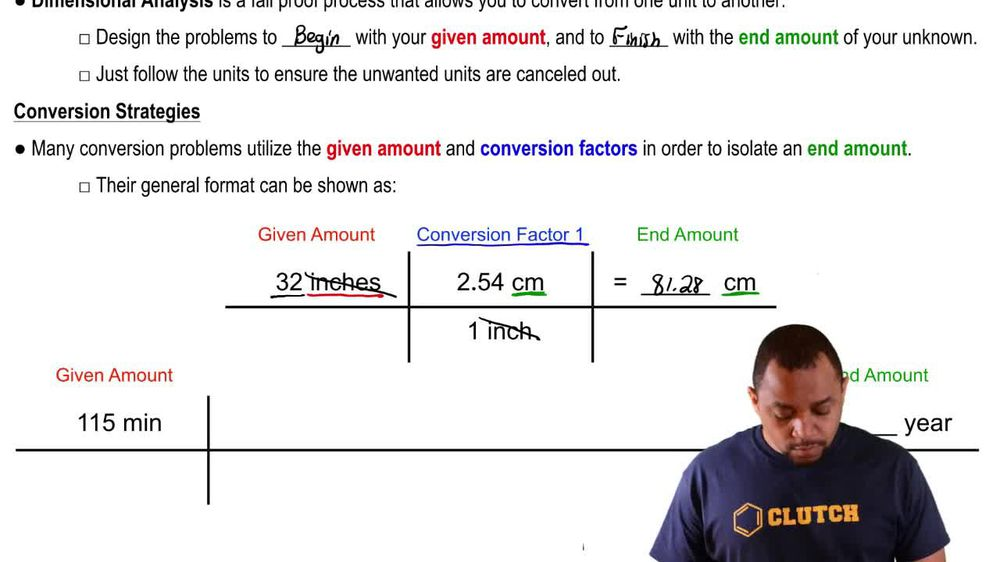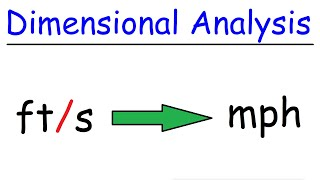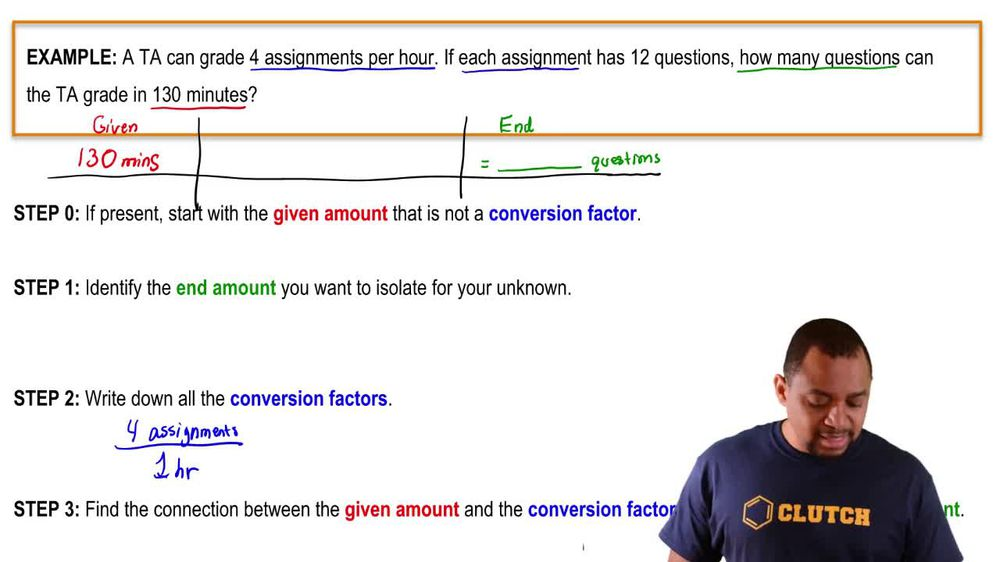Start typing, then use the up and down arrows to select an option from the list.
1. 1. Intro to General Chemistry2. Dimensional Analysis
Problem

# Gold can be hammered into extremely thin sheets called gold leaf. An architect wants to cover a 30 m * 25 m ceiling with gold leaf that is twelve-millionths of a centimeter thick. The density of gold is 19.32 g>cm3, and gold costs \$1654 per troy ounce 11 troy ounce = 31.1034768 g2. How much will it cost the architect to buy the necessary gold?

Relevant Solution3m
Play a video:
Hey everyone. So here it says a 90 ft by 72 ft ceiling is to be covered by gold leaf. A gold leaf is an extremely thin sheet of hammered gold, that is seven millions of an inch thick if gold costs \$995 per troy ounce. And remember the conversion factor for troy instagram's given, how much will it cost to cover the ceiling with gold leaf? Note the density of gold is 19.32 g per centimeter skewed. Alright, so realize here they're giving us feet feet and then inches here we need all the units to match. So we're gonna convert the feet into inches. We'll see later on why that is So remember that one ft is equal to 12 inches, right? So that's gonna come out to 10 80 inches here And then we have 72 ft And that's one ft is 12" as well. So that comes out to 864". Now that I have everything in inches, I can multiply them all together to get inches cute. So then here we're gonna multiply each of these together And then seven millions is 7.0 times 10 to the -6. And this is also one Here, we're going to multiply them all together and when we do that we're gonna get 6.53184", cute. Now the density given to us is in centimeters cubed. So what I should do next is convert inches cubed, two centimeters cubed. Now remember the conversion factor here is that 1" is equal to 2.54 cm. And since inches is cubed here we should cube the centimeters as well. And when we do that we're gonna get here 107.038 cm cubed. So we're gonna take that centimeters cubed And we're going to multiply by the density which is 19.32 g per one cm cubed. That comes out to be 2067. grants. And now that we have that we can convert that into actual real world dollar amount. The conversion factor is that one troy ounce which is abbreviated T. O. Is 31. g. And remember that one troy ounce we're told is \$995. So here basically to cover that entire ceiling The price will come out to be 66,000 154 and about 47 cents. So that would be our final answer. And if we look at our choices, that means our answer would have to be option B. So option B would be the cost of paying that whole ceiling in gold leaf